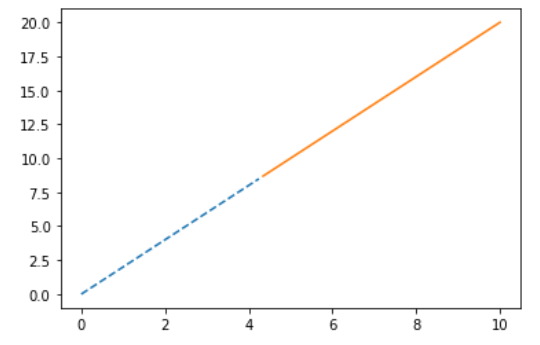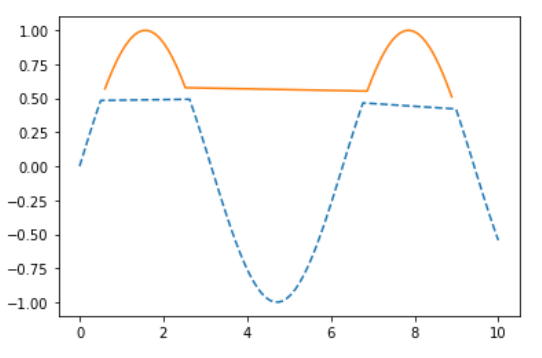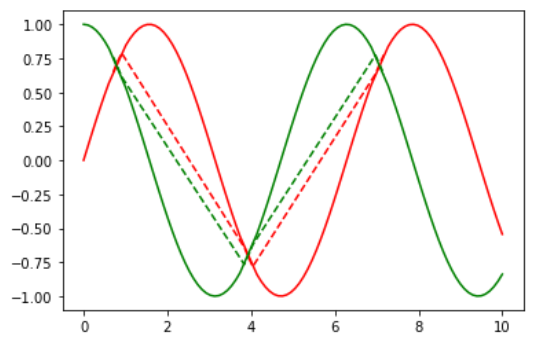Related Articles
Change matplotlib line style in mid-graph
• Last Updated : 12 Nov, 2020

Prerequisite: Matplotlib

In this article we will learn how to change line style in mid-graph using matplotlib in Python.

• Matplotlib: It is a tremendous visualization library in Python for 2D plots of arrays. Matplotlib may be a multi-platform data visualization library built on NumPy arrays and designed to figure with the broader SciPy stack. It was introduced by John Hunter within the year 2002.
• Line style: Line style is a feature that describes in which fashion or style line is drawn. Following image shows the key that has to provided as input parameter and what line style it will produce:Approach:

1. Import the matplotlib.pyplot library and other for data (optional)
2. Import or create some data
3. Draw a graph plot with different line style is middle.

Example 1:

In this example we will use simple steps mentioned above and form a graph with two different line styles.

## Python3

 `# importing packages``import` `matplotlib.pyplot as plt``import` `numpy as np`` ` `# create data``x ``=` `np.linspace(``0``, ``10``, ``100``)``y ``=` `3` `*` `x ``+` `2`` ` `below ``=` `y < ``15``above ``=` `y >``=` `15`` ` `# Plot lines below as dotted-------``plt.plot(x[below], y[below], ``'--'``)`` ` `# Plot lines above as solid________``plt.plot(x[above], y[above], ``'-'``)`` ` `plt.show()`

Output :Example 2 :

In this example we will use simple steps mentioned above and form a graph with two different line styles in one sine function.

## Python3

 `# importing packages``import` `matplotlib.pyplot as plt``import` `numpy as np`` ` `# create data``x ``=` `np.linspace(``0``, ``10``, ``100``)``y ``=` `np.sin(x)`` ` `below ``=` `y < .``5``above ``=` `y >``=` `.``5`` ` `# Plot lines below as dotted-------``plt.plot(x[below], y[below], ``'--'``)`` ` `# Plot lines above as solid_______``plt.plot(x[above], y[above], ``'-'``)`` ` `plt.show()`

Output :Example 3 :

This is similar to above example with extra cosine function to show different feature of line styles in mid graph.

## Python3

 `# importing packages``import` `matplotlib.pyplot as plt``import` `numpy as np`` ` `# create data``x ``=` `np.linspace(``0``, ``10``, ``100``)``y1 ``=` `np.sin(x)``y2 ``=` `np.cos(x)`` ` `below ``=` `abs``(y1``-``y2) < .``2``above ``=` `abs``(y1``-``y2) >``=` `.``2`` ` `# Plot lines below as dotted-------``plt.plot(x[below], y1[below], ``'r--'``)`` ` `# Plot lines below as dotted-------``plt.plot(x[below], y2[below], ``'g--'``)`` ` `# Plot lines above as solid_______``plt.plot(x[above], y1[above], ``'r-'``)`` ` `# Plot lines above as solid_______``plt.plot(x[above], y2[above], ``'g-'``)`` ` `plt.show()`

Output :Attention geek! Strengthen your foundations with the Python Programming Foundation Course and learn the basics.

To begin with, your interview preparations Enhance your Data Structures concepts with the Python DS Course.

My Personal Notes arrow_drop_up